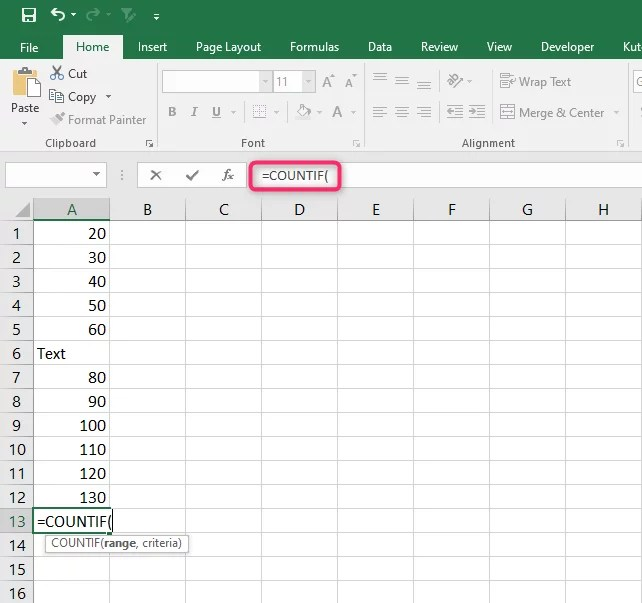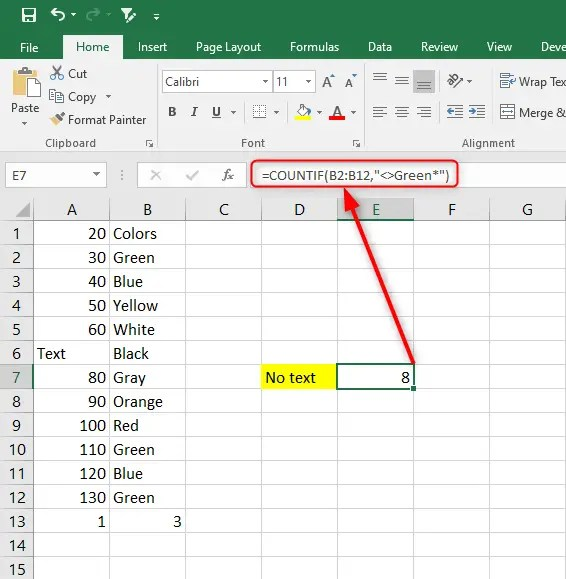# How to count Excel cells with text (with examples)

Microsoft is a great program that allows users to store and analyze large and even small amounts of data for interpretation. Apart from using only numerical values in Excel, you can also have cells that contain text within your data. To understand your data better, you need to differentiate cells that contain numbers and those with text. It is easy to do this as Excel provides you with all the features to use. Here we discuss the different ways you can count Excel cells that contain text.

## Using the COUNTIF Function to count all cells with text

1. In an open Excel worksheet, click on a blank cell. Here is where you will insert your formula.

2. Type in your function =COUNTIF (range, criteria) in that cell.3. For range, select the cell range you want to check whether there is text. In place of Criteria, type “*” with the quotes. The asterisks ensure we match all text values. For

4. Press the Enter key to apply your formula.## How to count Excel cells that contain specific text

If you need to match any specific text value, instead of using the asterisk value, you can specify the criteria you want to use.  So, when counting specific text in cells in your Excel worksheet, your function will look like this

`=COUNTIF (range, "text")`

So, if you want to count the number of cells that contain the text green in cells B2 through B12, here is what your formula will look like:

` =COUNTIF (B2:B12, “*Green*”).`

The asterisks symbol ensures that only the cells containing the text “green” with cells between B2 and B12 are returned.## How to count Excel cells that do not contain specific Text

For instance, you can use (<>) to count all cells that do not contain the text. So, when you use such a formula, here is what it will look like:

```=COUNTIF (range, “<>*”)
```The logical operator <> stands for not equal to as it counts non-text cells.

## Using SUMPRODUCT Function to count cells with any text in Excel

You can use the SUMPRODUCT and ISTEXT functions to get the number of cells that contain text in your worksheet. The SUMPRODUCT function ensures a logical test is performed on a range, then counts the results. The ISTEXT function returns the TRUE value when given a text value. It checks if each cell in the specified range contains any text characters. Here is how your function will be:

`=SUMPRODUCT (--ISTEXT (range))`

You can also choose to use this formula:

`=SUMPRODUCT (ISTEXT (range)*1)`

You can also use the SUMPRODUCT, ISNUMBER, and FIND functions in case-sensitive cases to find specific text. The three formulas when applied in a formula will look like this:

`=SUMPRODUCT (--(ISNUMBER (FIND (“text” range))))`

Example

`=SUMPRODUCT( -- (ISNUMBER(FIND("Green",B1:B12))))`The function combinations are explained:

The FIND function will search the specific text in the defined cell. It will also return the number of the starting position of the text used as criteria.

The ISNUMBER function ensures that you do not get an error as an answer. It is used as it helps avoid getting errors! as an answer whenever the FIND function does not match the defined criteria.